﻿ 基于CFD的燃烧轻气炮发射过程仿真
 舰船科学技术2022, Vol. 44Issue (10): 180-184    DOI: 10.3404/j.issn.1672-7649.2022.10.039PDF

Simulation for launch process of combustion light gas gun based on CFD
HU Bin, WANG Zhen, HE Hang, PENG Song-jiang
The 713 Research Institute of CSSC, Zhengzhou 450015, China
Abstract: Aiming at the internal ballistic control problem in the firing process of combustion light gas gun, the 19-step detailed chemical reaction mechanisms of burning hydrogen-oxygen were combined with the basic model of turbulent flow, considering the influence of both chemical kinetics and turbulence effect. Then numerical simulation calculations were carried out to analyze the influence of different initial conditions on the interior ballistic characteristics of the combustion light gas gun. The results show that the hydrogen-oxygen combustion in the chamber was in the form of turbulent flame combustion, the initial temperature and pressure can significantly affect the hydrogen-oxygen combustion process and change the interior ballistic performance. The volume of combustion chamber has great influence on energy utilization when the gas filling density in chamber remains unchanged. These simulation results provide a theoretical basis for further research of the combustion light gas gun.
Key words: turbulent combustion     CFD     interior ballistics     combustion light gas gun
0 引　言

1 模型描述 1.1 计算模型与网格设置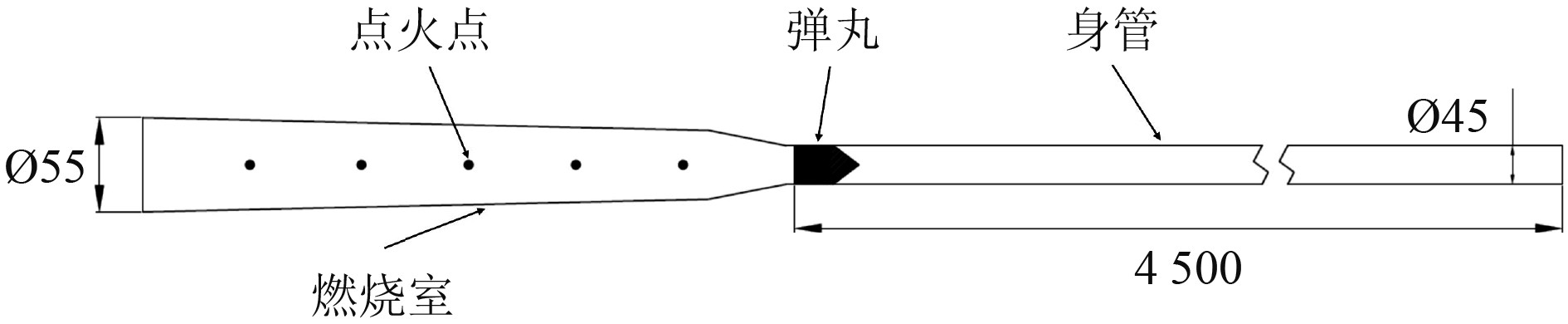图 1 燃烧轻气炮基本结构简化模型 Fig. 1 The simplified structure model of combustion light gas gun
1.2 流动控制方程

 $\frac{{\partial \rho }}{{\partial t}} + \frac{\partial }{{\partial \mathop \chi \nolimits_j }}\left( {\mathop {\rho \upsilon }\nolimits_j } \right) = 0,$ (1)
 $\frac{\partial }{{\partial t}}\left( {\rho \mathop \upsilon \nolimits_i } \right) + \frac{\partial }{{\partial \mathop \chi \nolimits_j }}\left( {\rho \mathop \upsilon \nolimits_i \mathop \upsilon \nolimits_j + \frac{2}{3}\mathop \delta \nolimits_{ij} \rho k} \right) = - \frac{{\partial p}}{{\partial \mathop \chi \nolimits_i }} + \frac{{\partial \mathop \tau \nolimits_{ij} }}{{\partial \mathop \chi \nolimits_j }},$ (2)
 $\frac{\partial }{{\partial t}}\left( {\rho E} \right) + \frac{\partial }{{\partial \mathop \chi \nolimits_j }}\left( {\rho \mathop \upsilon \nolimits_j H} \right) = \frac{\partial }{{\partial \mathop \chi \nolimits_j }}\left( {\rho \mathop a\nolimits_{eff} \frac{{\partial H}}{{\partial \mathop \chi \nolimits_j }} + \mathop \tau \nolimits_{ij} \mathop \upsilon \nolimits_i } \right) ,$ (3)
 $\frac{\partial }{{\partial t}}\left( {\rho \mathop Y\nolimits_k } \right) + \frac{\partial }{{\partial \mathop \chi \nolimits_j }}\left( {\rho \mathop Y\nolimits_k \mathop u\nolimits_j } \right) = \frac{\partial }{{\partial \mathop x\nolimits_j }}\left( {\rho \mathop D\nolimits_{eff} \frac{{\partial \mathop Y\nolimits_k }}{{\partial \mathop \chi \nolimits_j }}} \right) + \mathop {\dot \omega }\nolimits_{_k}。$ (4)

1.3 湍流模型

 $\begin{split} \frac{{\partial \left( {\rho k} \right)}}{{\partial t}} + \frac{{\partial \left( {\rho k\mathop u\nolimits_i } \right)}}{{\partial \mathop \chi \nolimits_i }} = &\frac{\partial }{{\partial \mathop \chi \nolimits_j }}\left[ {\left( {\mu + \frac{{\mathop \mu \nolimits_t }}{{\mathop \sigma \nolimits_k }}} \right)\frac{{\partial k}}{{\partial \mathop \chi \nolimits_j }}} \right] +\\ & \mathop G\nolimits_k + \mathop G\nolimits_b - \rho \varepsilon - \mathop Y\nolimits_M + \mathop S\nolimits_k , \end{split}$ (5)
 $\begin{split} \frac{{\partial \left( {\rho \varepsilon } \right)}}{{\partial t}} +& \frac{{\partial \left( {\rho \varepsilon \mathop u\nolimits_i } \right)}}{{\partial \mathop \chi \nolimits_i }} = \frac{\partial }{{\mathop {\partial \chi }\nolimits_j }}\left[ {\left( {\mu + \frac{{\mathop \mu \nolimits_t }}{{\mathop \sigma \nolimits_\varepsilon }}} \right)\frac{{\partial \varepsilon }}{{\partial \mathop \chi \nolimits_j }}} \right] + \\ & \mathop C\nolimits_{1\varepsilon } \frac{\varepsilon }{k}\left( {\mathop G\nolimits_k +\mathop C\nolimits_{3\varepsilon } \mathop G\nolimits_b } \right) - \mathop C\nolimits_{2\varepsilon } \rho \frac{{\mathop \varepsilon \nolimits^2 }}{k} + \mathop S\nolimits_\varepsilon。\end{split}$ (6)

1.4 燃烧模型

 $w = \mathop k\nolimits_0 \mathop T\nolimits^b \mathop e\nolimits^{ - E/RT} \left[ A \right]\left[ B \right]。$ (7)表 1 H2/O2 燃烧机理 Tab.1 The combustion mechanism of H2/O2
2 计算结果与分析 2.1 模型验证表 2 45 mm燃烧轻气炮试验与计算结果对比 Tab.2 The comparison of test and calculation results of 45 mm combustion light gas gun
2.2 氢氧燃烧过程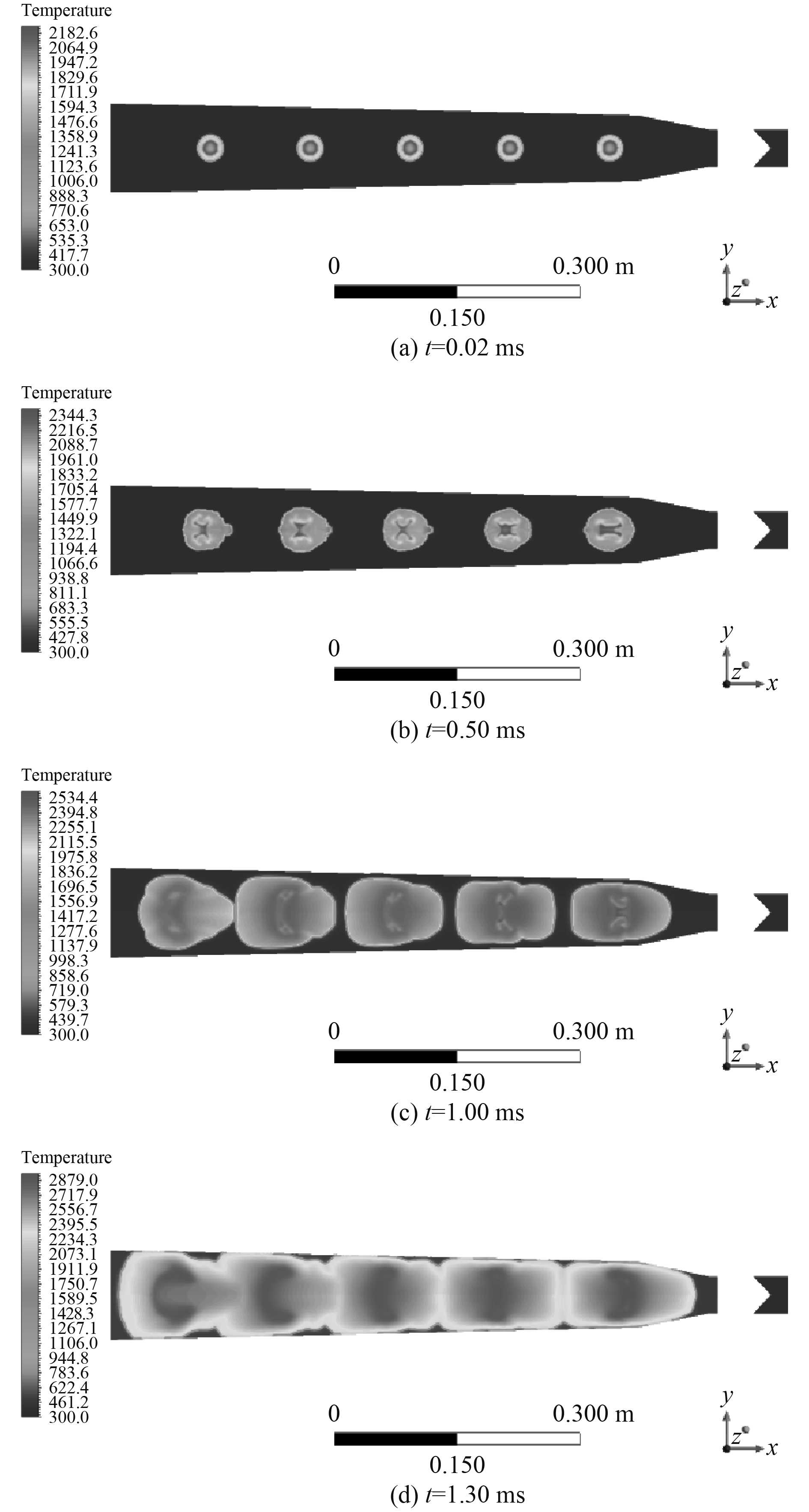图 2 初始阶段温度变化云图 Fig. 2 The cloud image of temperature in initial stage
2.3 初始温度对内弹道性能的影响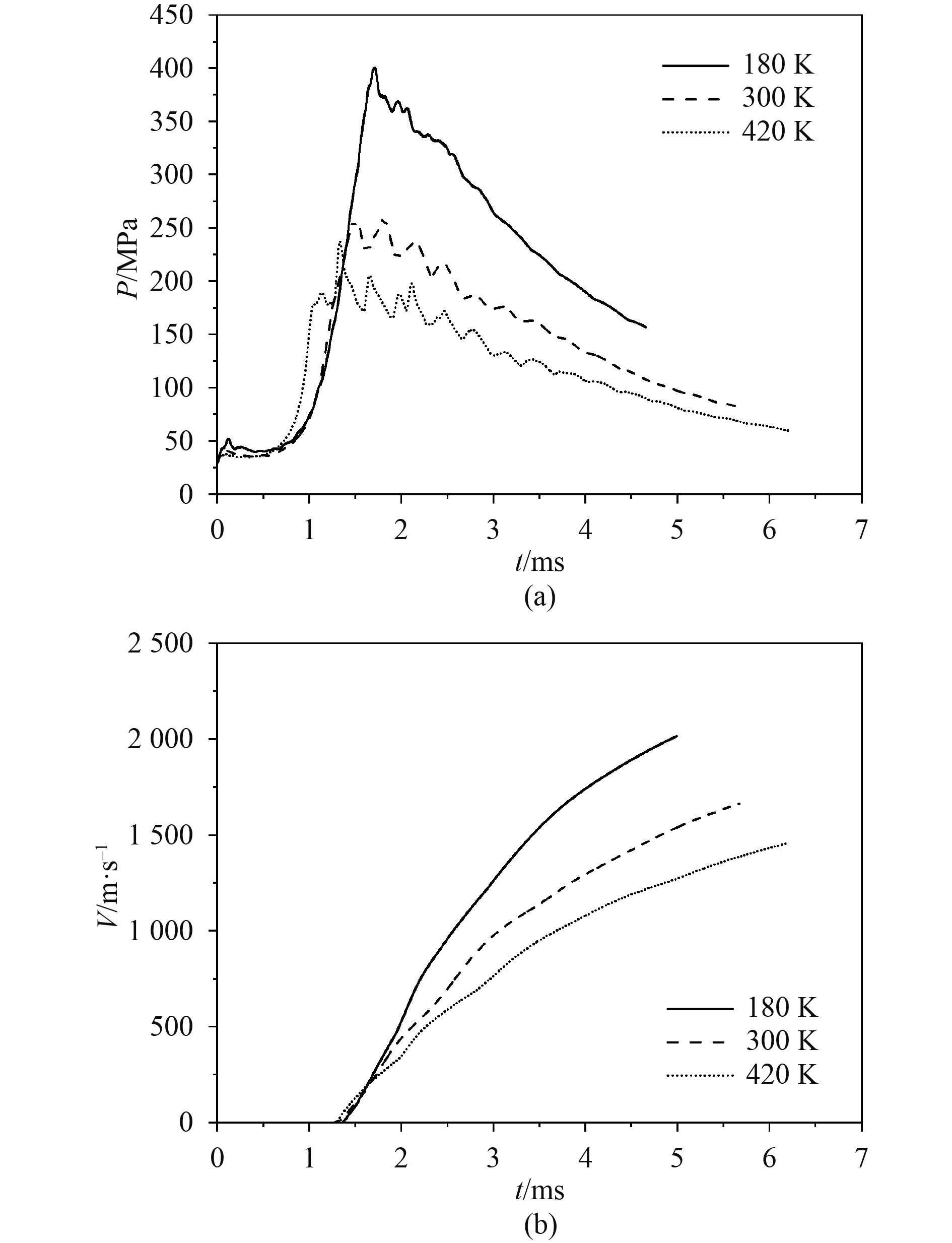图 3 不同初始温度对膛压和弹丸速度的影响 Fig. 3 The influence of different initial temperatures on chamber pressure and muzzle velocity
2.4 初始压力对内弹道性能的影响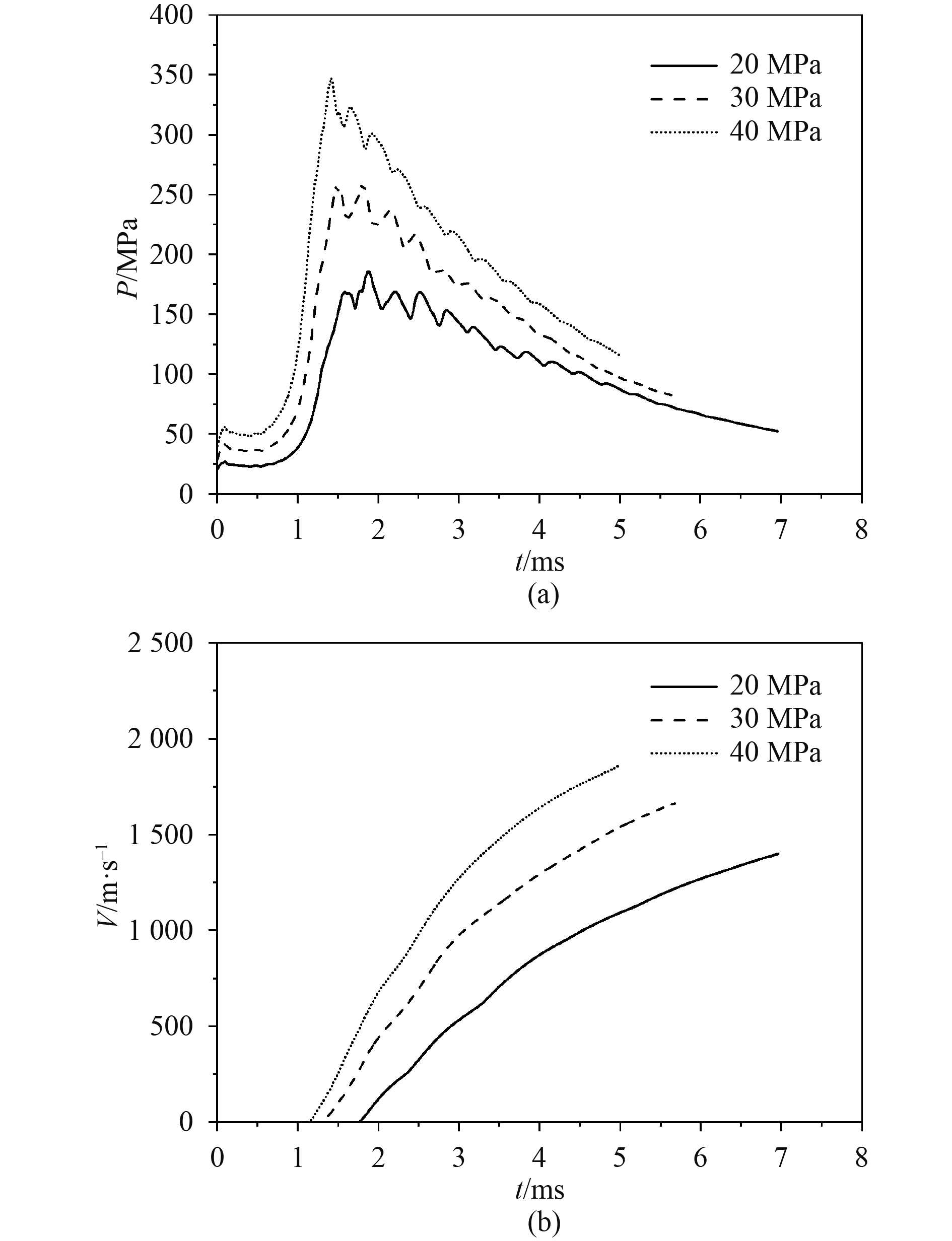图 4 不同初始压力对膛压和弹丸速度的影响 Fig. 4 The influence of different initial pressures on chamber pressure and muzzle velocity
2.5 燃烧室容积对内弹道性能的影响表 3 不同燃烧室容积计算结果对比 Tab.3 The calculation results comparison of different combustion chamber volumes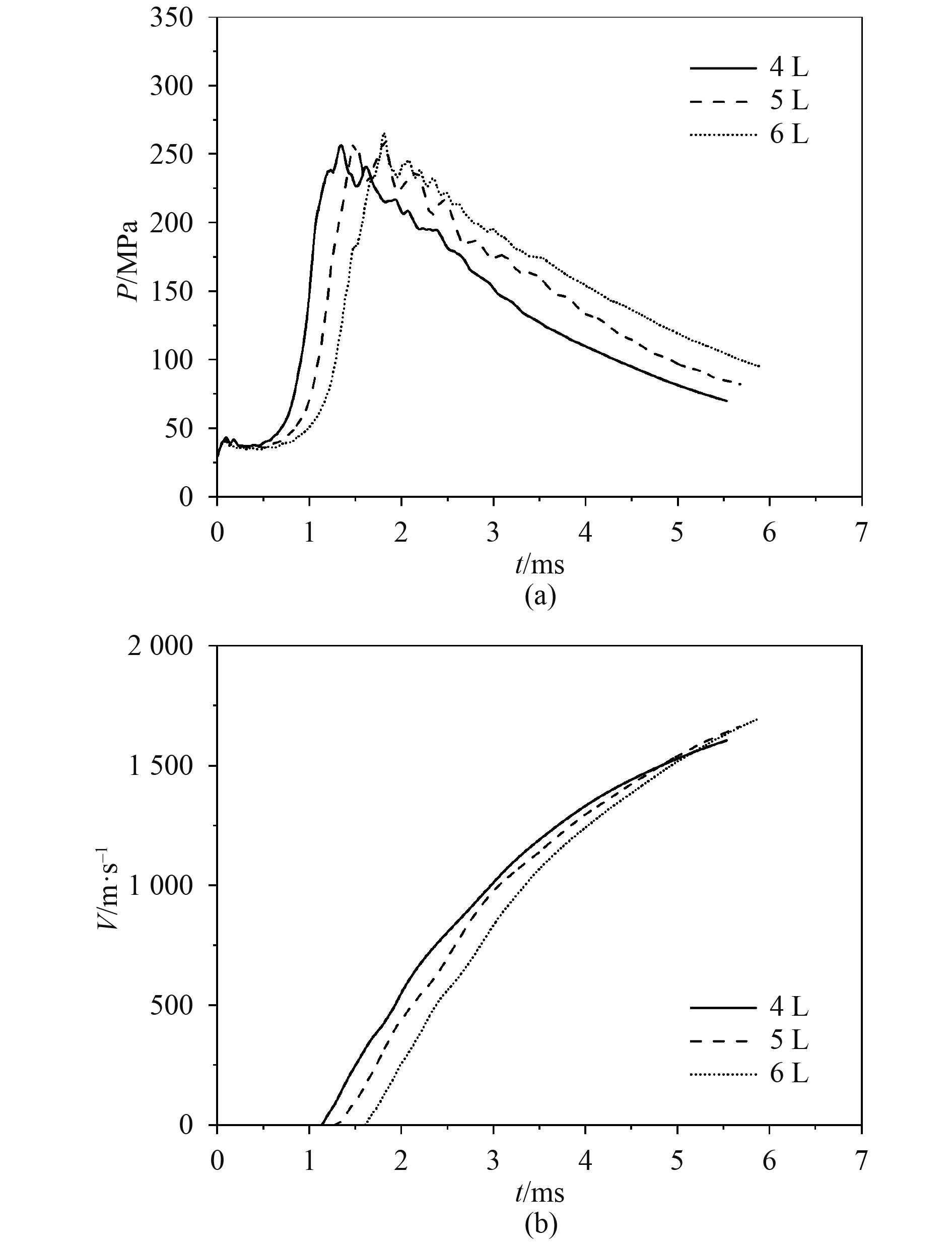图 5 不同燃烧室容积对膛压和弹丸速度的影响 Fig. 5 The influence of different chamber volumes on chamber pressure and muzzle velocity
3 结　语

1）膛内氢氧燃烧呈现出湍流火焰特征，随着燃烧火焰的增大，湍流作用加剧，火焰传播速度加快；

2）初始温度对氢氧燃烧过程影响较大，初始温度降低，氢氧发射药总能量增大，膛压增大，压力波动减小，弹丸初速增加；

3）初始压力增大时，发射药总能量增加，膛压增大，弹丸初速增大，实际应用中应该合理地选择发射药气体的初始装填压力，避免膛压过高；

4）保持装填气体密度不变，扩展燃烧室容积可以在一定程度上提高弹丸初速，但是能量利用率降低，做功能力下降。

  于子平, 张相炎. 新概念火炮[M]. 北京: 国防工业出版社, 2012.  胡静, 张明安, 岳文龙, 等. 内弹道过程中气体工质做功能力计算分析[J]. 火炮发射与控制学报, 2011, (6): 82-85.  TIDMAN D A, MASSEY D W, WITHERSPOON F D, et al. Compact light gas gun firings with high pressure gaseous and solid energetic materials - experiments and analysis[C]// 31st JANNAF Combustion Subcommittee Meeting, Sunnyvale, CA, 17-21 Oct, 1994.  KRUCZYNSKI D, MASSEY D. Combustion light gas gun technology demonstration[R]. ADA462130, 2007.  邓飞, 张相炎. 燃烧轻气炮氢氧燃烧特性详细反应动力学模拟[J]. 兵工学报, 2014, 35(3): 415-420. DENG F, ZHANG X Y. Simulation of hydrogen-oxygen combustion of combustion light gas gun using detailed chemical kinetics model[J]. Acta Armamentarii, 2014, 35(3): 415-420. DOI:10.3969/j.issn.1000-1093.2014.03.019  黄滔, 张相炎, 刘宁. 燃烧轻气炮点火对内弹道性能影响的仿真分析[J]. 兵工自动化, 2014, 33(12): 25-28. HUANG T, ZHANG X Y, LING N. Simulation analysis on influence of ignition on internal ballistics of combustion light gas gun[J]. Ordnance Industry Automation, 2014, 33(12): 25-28. DOI:10.7690/bgzdh.2014.01.008  ZHOU Fei, LIU Ning, ZHANG Xiangyan, et al. 1D study of the detonation phenomenon and its influence on the interior ballistics of the combustion light gas gun[J]. Defence Technology, 2020, 16(2): 341-347. DOI:10.1016/j.dt.2019.06.009  孙从煌, 曲艳东, 刘万里, 等. 点火条件对密闭管道内预混氢气/空气燃爆特性的影响[J]. 爆炸与冲击, 2018, 38(3): 622-631. SUN C H, QU Y D, LIU W L, et al. Influence of different ignition conditions on deflagration characteristics of a premixed mixture of H2 and air in a closed pipe [J]. Explosion and shock waves, 2018, 38(3): 622-631.  MUELLER M A, KIM T J, YETTER R A, et al. Flow reactor studies and kinetic modeling of the H2/O2 reaction [J]. International Journal of Chemical Kinetics, 1999, 31(2): 113-125. DOI:10.1002/(SICI)1097-4601(1999)31:2<113::AID-KIN5>3.0.CO;2-0  刘玉坪, 肖民, 黄志伟, 等. 基于化学反应动力学的双燃料发动机数值模拟[J]. 舰船科学技术, 2021, 43(6): 122–129. LIU Y P, XIAO M, HUANG Z W, et al. Numerical simulation of dual fuel engine based on chemical reaction mechanism, 2021, 43(6): 122–129.